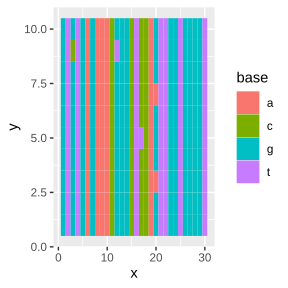# substitution_models

## Substitution models

NodeSub includes many different functions to generate alignments, this file serves to provide an overview of the different models. The standard alignment function is given by ‘sim_normal’, which is based on the alignment simulation functions in the package phangorn.

seq_length <- 30
sub_rate <- 1 / seq_length

input_tree <- TreeSim::sim.bd.taxa(n = 10,
numbsim = 1,
lambda = 1,
mu = 0.1,
complete = TRUE)[]

normal_alignment <- sim_normal(input_tree,
l = seq_length,
rate = sub_rate)

plot_phyDat(normal_alignmentalignment)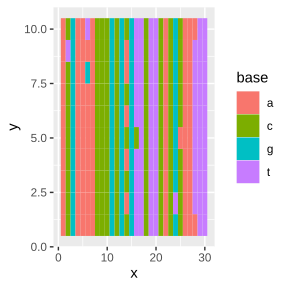Then, there are two node substitution models available, the unlinked and the linked model. In the unlinked model, both daughter branches accumulate substitutions independently from each other during speciation. In the linked model, the substitutions in the daughter branches are conditional on each other, such that substitutions accumulated in one daughter, are not able to be accumulated in the other daughter. For both models we need to specify the node time (tau). For the linked model rates are specified slightly differently, with the substitution rate reflecting the rate at which one of the daughters accumulates a substitution, and the node_mut_rate_double reflecting the rate at which both daughters accumulate a (different) substitution. unlinked_alignment <- sim_unlinked(input_tree, rate1 = sub_rate, rate2 = sub_rate, l = seq_length, node_time = 0.5) plot_phyDat(unlinked_alignmentalignment)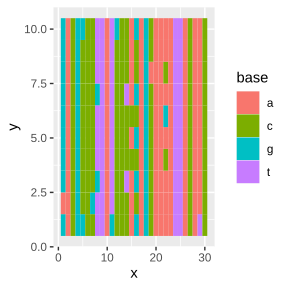linked_alignment <- sim_linked(input_tree,
rate = sub_rate,
node_mut_rate_double = sub_rate * sub_rate,
node_time = 0.5,
l = seq_length)

plot_phyDat(linked_alignmentalignment)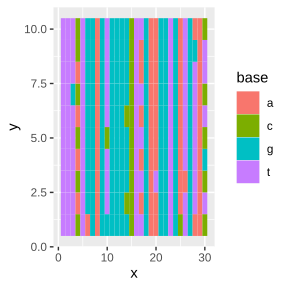# Explicit models The linked and unlinked alignment simulators use Markovian mathematics to calculate the expected number of substitutions, which yields the correct mutations along a branch, but which neglects any ‘reverse’ mutations (as these are masked). If the need arises to more explicitly simulate the mutational process, we have provided explicit functions for both normal and the unlinked model: unlinked_explicit <- sim_unlinked_explicit(input_tree, rate1 = sub_rate, rate2 = sub_rate, l = seq_length, node_time = 0.5) plot_phyDat(unlinked_explicitalignment)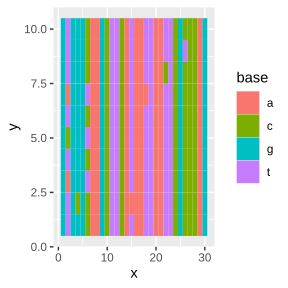normal_explicit <- sim_normal_explicit(input_tree,
l = seq_length,
rate = sub_rate)

plot_phyDat(normal_explicit\$alignment)